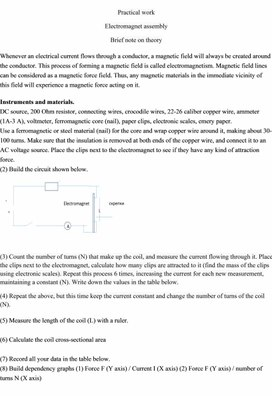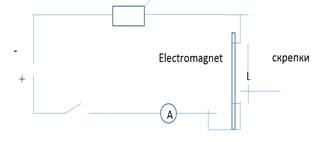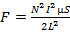PHY_10_60_V1_DM_Artificial magnets. Solenoid
Оценка 5

# PHY_10_60_V1_DM_Artificial magnets. Solenoid

Оценка 5
docx
08.05.2020PHY_10_60_V1_DM_Artificial magnets. Solenoid.docx

Practical work

Electromagnet assembly

Brief note on theory

Whenever an electrical current flows through a conductor, a magnetic field will always be created around the conductor. This process of forming a magnetic field is called electromagnetism. Magnetic field lines can be considered as a magnetic force field. Thus, any magnetic materials in the immediate vicinity of this field will experience a magnetic force acting on it.

`Instruments and materials.`
`DC source, 200 Ohm resistor, connecting wires, crocodile wires, 22-26 caliber copper wire, ammeter (1A-3 A), voltmeter, ferromagnetic core (nail), paper clips, electronic scales, emery paper.`
`Use a ferromagnetic or steel material (nail) for the core and wrap copper wire around it, making about 30-100 turns. Make sure that the insulation is removed at both ends of the copper wire, and connect it to an AC voltage source. Place the clips next to the electromagnet to see if they have any kind of attraction force.`
`(2) Build the circuit shown below.`(3) Count the number of turns (N) that make up the coil, and measure the current flowing through it. Place the clips next to the electromagnet, calculate how many clips are attracted to it (find the mass of the clips using electronic scales). Repeat this process 6 times, increasing the current for each new measurement, maintaining a constant (N). Write down the values ​​in the table below.

(4) Repeat the above, but this time keep the current constant and change the number of turns of the coil (N).

`(5) Measure the length of the coil (L) with a ruler.`
` `
`(6) Calculate the coil cross-sectional area`
` `
`(7) Record all your data in the table below.`
`(8) Build dependency graphs (1) Force F (Y axis) / Current I (X axis) (2) Force F (Y axis) / number of turns N (X axis)`

Result table

 Current I/А `Number of screws` Number of slides The mass scrap Clip weight Cross sectional area Coil length

(9) Using forceand   P= F,
determine the mean value of the magnetic constant
µ

Mean value µ =  ----------------------------------------------

Conclusion:

Скачать файл